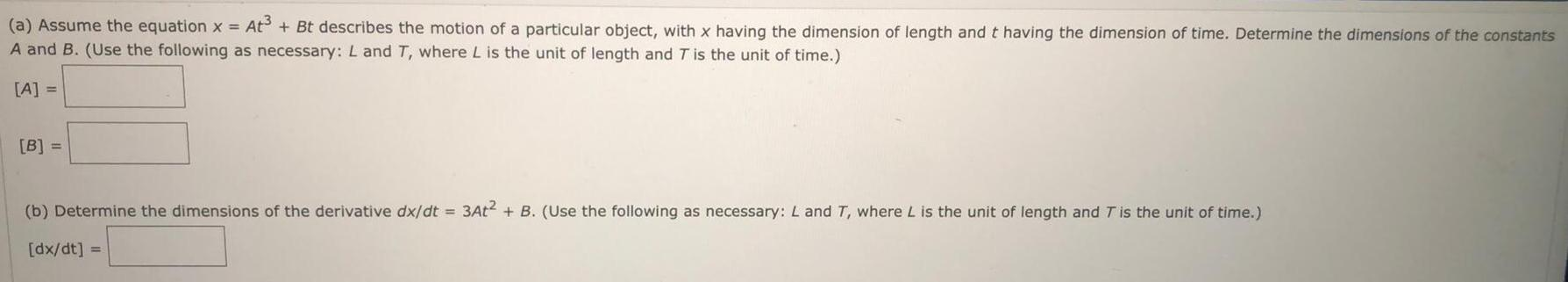Question:

# (a) Assume the equation x = At³ + Bt describes the motion of

Last updated: 7/30/2022(a) Assume the equation x = At³ + Bt describes the motion of a particular object, with x having the dimension of length and t having the dimension of time. Determine the dimensions of the constants A and B. (Use the following as necessary: L and T, where L is the unit of length and T is the unit of time.) [A] = [B] = (b) Determine the dimensions of the derivative dx/dt = 3At² + B. (Use the following as necessary: L and T, where L is the unit of length and T is the unit of time.) [dx/dt] =